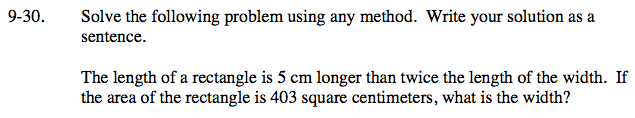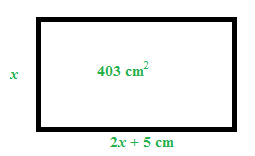### Home > CCA > Chapter 9 > Lesson 9.1.3 > Problem9-30

9-30.

Solve the following problem using any method. Write your solution as a sentence.

The length of a rectangle is 5 cm longer than twice the length of the width. If the area of the rectangle is 403 square centimeters, what is the width? Homework Help ✎Make a diagram and label the sides.

Write an equation to solve for the width (x).

Distribute and solve for x.

Use the quadratic formula to solve for x.

$x=\frac{52}{4}=13 \;\;\;\;\;\;\;\;\; x=\frac{-62}{4}=-15.5$

Can't have a negative length.(2x + 5)(x) = 403

2x2 + 5x = 403
2x2 + 5x − 403 = 0

$x=\frac{-5\pm\sqrt{5^{2}-4(2)(-403)}}{2(2)}$

$x=\frac{-5+57}{4} \; \text{and} \; x=\frac{-5-57}{4}$